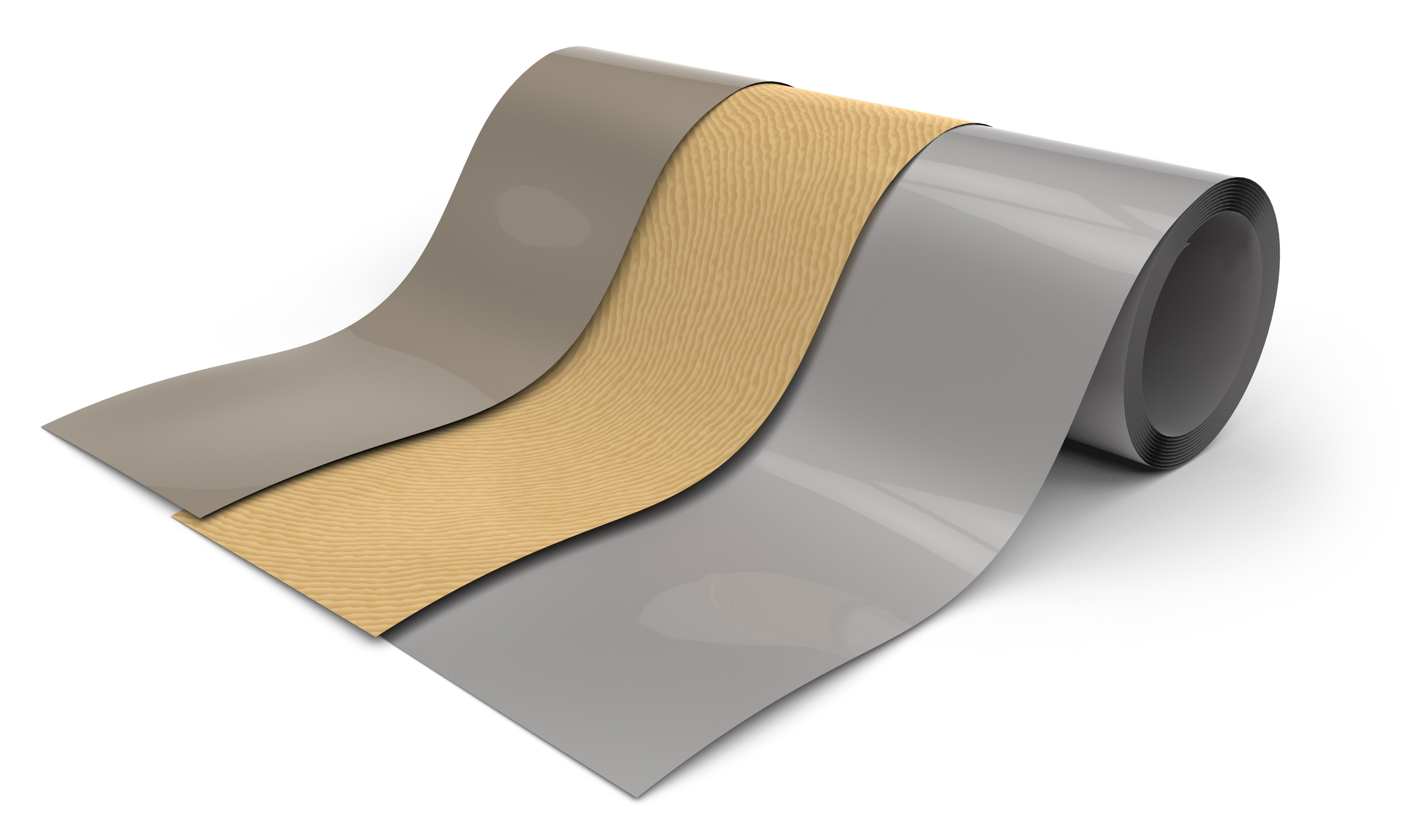Susanna Laurén Mar 5, ’19 ~ 3 min

# Which surface free energy method should be used?

## Surface Science Blog

OWRK, Wu, Acid-base, Equation of state… You might find it confusing when seeing the names of all the different methods for surface free energy calculations. There are however some clear differences and similarities between them. As surface free energy can give you more information on your surface compared to the contact angle measurements alone, it is time well spent to study the different theories in more detail.

## Surface free energy is typically divided to polar and dispersive parts

One of the main differences between the theories is how the molecular interactions are treated. The methods can be divided into single and multicomponent approaches. The equation of state and Zisman are the most commonly used single component methods. More commonly the surface free energy is divided into different components; polar and dispersive. This is used in theories, like OWRK and Wu. The acid-base theory goes even further by dividing the polar component into acid and base components.

## Different surface free energy theories to serve different needs

Different theories have been developed because there has been a need for a new method to better describe the problem at hand. The same problem can be described with different mathematical models. For example, the difference between OWRK and Wu is in mathematics how the molecular interactions are treated. OWRK uses the geometric mean whereas Wu realizes on the harmonic one. Using harmonic mean gives little bit more emphasis on the polar interaction than the geometric one.

The acid-base method was developed to study biological systems and is thus the most used method in biomaterial research. It takes into account that many biopolymers are monopolar, meaning that they have only electron-acceptor (acid) or electron-donor (base) part.

Then there are special cases that need theories of their own. Schultz methods are developed to study high surface energy surfaces, that are difficult to measure in the air as contact angles on them tend to be low. The measurements are done in liquid instead which need to be taken into account in the equations used.## Explore the blog

You have only scratched the surface.